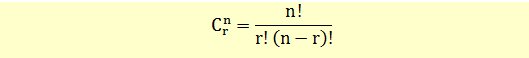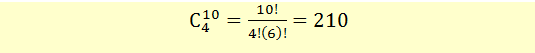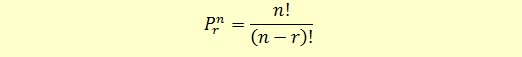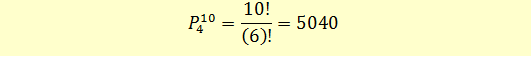# Permutation and Combination Formula

### Combination Formula

This is a special case of multinomial formula where the types of labels k=2. This means that the n objects can be labelled only in two ways and n1 + n2 = n.

For example, suppose we had to label 4 of our 10 stocks as BUY and the remaining 6 as SELL.

So, n=10, n1 = 4 and n2 = 6.

Let’s say n1 = r = 4, in that case n2 can be rewritten as n2 = n – r or 10 – 4 = 6

We can rewrite our formula as follows:This is called the combination formula and is read as n combination r, i.e., how many ways can we select a group of size r from a group of n objects.The combination problems can be solved directly on your BA II Plus calculator using the nCr function.

The Combination formula has its application in binomial trees.

### Permutation Formula

Note that in combinations, the order in which the objects are listed does not matter, that is A, B is the same as B, A. However, there could be a situation where the order matters. For example, from our group of 10 stocks, we want to select 4 stocks and rank them as No. 1, 2, 3, and 4. To solve this problem, we need to use the permutation formula which accounts for ordering of objects.This is read as the number of permutations of r objects from total n objects.

In our example:There are 5040 ways of selecting 4 objects from a group of 10 objects when ordering of objects is important.

Get our Data Science for Finance Bundle for just $29$51.